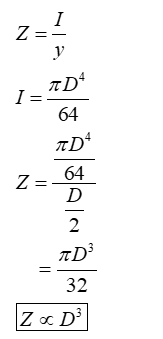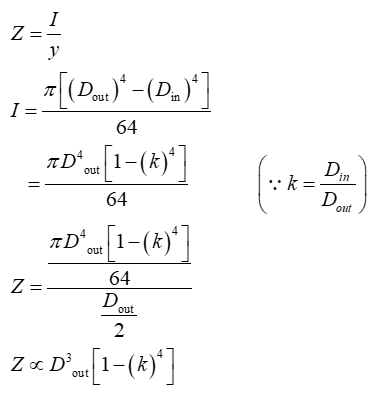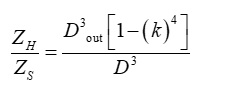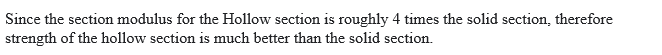# what-is-the-ratio-of-the-strength-of-a-solid-steel-column-of-150-mm-diameter-to-that-of-a-hollow-circular-steel-column-of-the-same-cross-sectional-area-and-a-wall-thickness-of-15-mm-the-two-columns

The given problem can be solved by using buckling criteria and rigidity criteria as well, but in the problem ends condition is not provided therefore go far the strength rigidity criteria, however, both criteria would have the same result.

Section Modulus,
The section modulus of the cross-sectional shape is of significant importance in designing beams. It is a direct measure of the strength of the beam. A beam that has a larger section modulus than another will be stronger and capable of supporting greater loads.
Find the diameter of the hollow section with the help of the given condition.Find the section modulus of the solid section.Now find the section modulus for the hollow section.Now find the section modulus for the hollow section.Now find the ratio of section modulus of both the section.Now substitute the required value in the above expression.